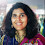## Saturday 7 June 2014

### Attitude

💠 Sharing this message that I received from my family-members & friends via Email & WhatsApp.

This is a relevant and popular message as many people have shared the same with me so far.
Do read if you are yet to do so...

Very interesting & meaningful message:

🔰

🔺If:
A B C D E F G H I J K LM N O P Q R S T U V W X Y Z
is equal to:
1 2 3 4 5 6 7 8 9 10 11 12 13 14 15 16 17 18 19 20 21 22 23 24 25 26

🔻Then,
🔹H+A+R+D+W+O+R+K;
8+1+18+4+23+15+18+11=98%

🔹K+N+O+W+L+E+D+G+E;
11+14+15+23+12+5+4+7+5=96%

🔹L+O+V+E;
12+15+22+5 = 54%

🔹L+U+C+K ;
12+21+3+11 = 47%

🔸None of them makes 100%. Then what makes 100%?

🔸Is it Money? NO!
🔹M+O+N+E+Y= 13+15+14+5+25=72%

🔹L+E+A+D+E+R+S+H+I+P= 12+5+1+4+5+18+19+8+9+16=97%

🔸Every problem has a solution, only if we perhaps change our "ATTITUDE"...

🔹A+T+T+I+T+U+D+E ;
1+20+20+9+20+21+4+5 = 💯%

It is therefore OUR ATTITUDE👍 towards Life and Work that makes
OUR Life 💯%Successful.

🔰
👌Amazing Mathematics🔣

Makes sense to take care of our Attitude!

Imagine if everyone takes care of their attitude what a great place Planet Earth will be!

1.1.